# Gate Mock Test- 14: CS/IT

## 65 Questions MCQ Test GATE Computer Science Engineering(CSE) 2023 Mock Test Series | Gate Mock Test- 14: CS/IT

Description
Attempt Gate Mock Test- 14: CS/IT | 65 questions in 180 minutes | Mock test for GATE preparation | Free important questions MCQ to study GATE Computer Science Engineering(CSE) 2023 Mock Test Series for GATE Exam | Download free PDF with solutions
QUESTION: 1

### Pipe A can fill a tank three times as fast as pipe B. If together two pipes can fill the tank in 48 min, the slower pipe alone will be able to fill the tank in:

Solution:

A = 3B

Ratio of efficiency, A : B = 3 : 1

Ratio of times, A : B = 1 : 3 Total capacity = Total efficiency × Total time = 4 × 48 = 192 unit Time taken by slower pipe

B = Total Capacity / Efficiency of B = 192 / 1 = 192 min

QUESTION: 2

### In the following question, out of the four alternatives, select the word opposite in meaning to the given word.Gratuitous

Solution: The word “gratuitous” means given or done free of charge. Thus, the word “costly” would be the correct antonym of the given word.

Gratis means without charge; free.

QUESTION: 3

### Find the area bounded between parabola and the line y2 = x2y = 2

Solution: y = 2 y2 = x

⇒ x = 22 = 4

: Parabola and line intersect at the point (4,2) ∴ Area =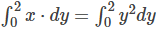⇒ A =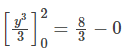= 8/3 sq.units

QUESTION: 4

Direction: The bar graph shows the number of employees working under the six different Departments (A, B, C, D, E, F) of a certain company. Study the diagram and answer the following questions.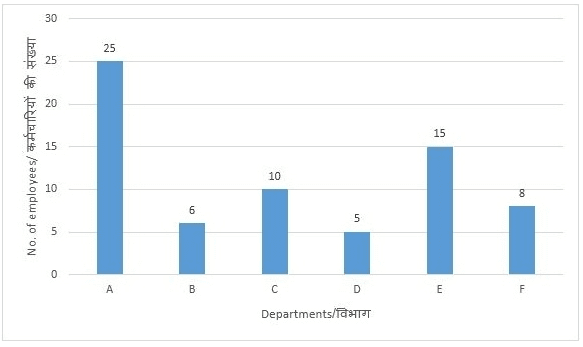If departments F and D are merged to create a new department G, then which department will have the least number of employees?

Solution: If departments F and D are merged to create a new department G, then

Employees in department A = 25

Employees in department B = 6

Employees in department C = 10

Employees in department E = 15

Employees in department G = 8

∴ Department B has the least number of employees.

QUESTION: 5

In the following question, a sentence is given with a blank to be filled in with an appropriate word. Select the correct alternative out of the four and indicate it by selecting the appropriate option.

Confusion prevails in madrasas in Uttar Pradesh over the distribution of free NCERT textbooks at the academic session ____________ from August.

Solution: The answer is ‘has begun’ because we use Present Perfect Tense, if the action is important and not the time of action or an action that has recently finished.
QUESTION: 6

A sum of Rs. 400 amounts to Rs. 480 in 4 years. What will it amount to if the rate of interest is increased by 2 % for the same time?

Solution:

We know that, S.I = P x R x T / 100

A = S.I + P

480 = 5.1+400

⇒S.I = 480 − 400 = 80

⇒S.I = P × R × T100

⇒80 = 400 × R × 4100

⇒R = 5%

Now rate is increased by 2% So, new rate is 7% New S.I=400 × 7 × 4 / 100 = Rs.112

New Amount = S.I + P = 112 + 400 = Rs.512

QUESTION: 7

The question below consists of a set of labelled sentences. These sentences, when properly sequenced form a coherent paragraph. Select the most logical order of sentences from among the options.

P: The Information and Broadcasting Ministry plans to conduct an independent study to gauge the

Q: The advertisements are carried on various platforms, including print and visual media.

R: The Directorate of Advertising and Visual Publicity (DAVP) is the nodal agency of the government

for advertising on behalf of the various ministries.

S: The initiative comes ahead of the Lok Sabha election in 2019 for which the government is expected

to reach out to the people and highlight the works done by it in the past 4 years.

Solution:

The paragraph talks about the plans and advertisements of The Information and Broadcasting Ministry, which is given in sentence P. P is the first statement. The word ‘initiative’ given in the sentence S is talking about the plans. Hence, S must follow P. Now, the introduction of the advertising agency is given in the sentence R, which must be the next statement. Thus, the sequence after rearrangement is PSRQ and option B is the correct answer.

QUESTION: 8

In the following question, some parts of the sentence may have errors. Find out which part of the sentence has an error and select the appropriate option. If the sentence is free from error, select 'No error'.

The gold foil used liberal (1)/ in Thanjavur paintings serves (2)/ many objectives that makes the painting more attractive. (3)/ No error

Solution:

The error is in part (1) of the sentence. Change ‘liberal’ to ‘liberally’ because in this sentence it is in adjective form while the proper usage of liberal is in its adverb form i.e. ‘liberally’ as it qualifies the gold foil here.

QUESTION: 9

A,B and C can do a job in 6 days, 12 days and 15 days respectively. C works till 1/8 of the work is completed and then leaves. Rest of the work is done by A and B together. Time taken to finish the remaining work by A \& B together is how much?

Solution:

Remaining work = 1 - 1/8 = 7 / 8

(A+B)′s1 day′s work =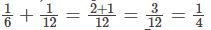∴ Time taken in doing 7/8 part of work = ⅞ x 4 = 7/2

=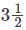days

QUESTION: 10

A invests 1/3 part of the capital for 1/6 of the time, B invests 1/4 part of the capital for 1/2 of the time and C invests the rest of the capital for the rest of the time. Out of a profit of Rs. 23000, B’s share is?

Solution:

Ratio of their investment,

=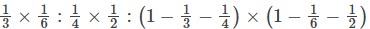=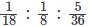=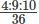= 4 : 9 : 10

B's share = 23000 x 9/23 = Rs. 9000

QUESTION: 11

Identify the correct translation into logical notation of the following assertion.

“You can not ride the roller coaster if you are under 4 feet tall unless you are older than 16 years old.”

Where q = “You can ride the roller coaster”

r = “You are under 4 feet tall”

s = “You are older than 16 years old”

Solution: A à B can be represented like B if A.

The third option seems the most appropriate as ~Q is on the right-hand side.

(r∧= s)→-q . If you try to interpret this mathematical statement, you will get that this is the most appropriate.

QUESTION: 12

The traditional computer system stores the data in the form of binary. Arjun is a professor who teaches binary systems to the students. He observes that if ternary (radix 3) is used instead of binary, space can be utilised more efficiently. If n be the no of bits in string when data is stored in the form of binary, then ternary system will need

Solution: Far a n bit string in binary, maximum data which can be

represented = 2n

No of bits required to stare it intaternary format= log3⁡2n = nlog3⁡2

QUESTION: 13

Consider a processor that includes a base register with indexing addressing mode. Suppose an instruction is encountered that employs this addressing mode and specifies a displacement of 7B2, in hexadecimal. Currently the base and index register contain the decimal numbers 48022 and 8, respectively. What is the address of the operand (In Decimal)?

Solution: Address of operand= base register value + Index Value + Displacement

= 48022 + 8+ 1970

= 50000

QUESTION: 14

A non-pipelined processor has a clock rate of 2.5 GHz and an average CPI (cycles per instruction) of 4. An upgrade to the processor introduces a five-stage pipeline. However, due to internal pipeline delays, such as latch delay, the clock rate of the new processor has to be reduced to 2 GHz.

What is the speedup achieved for executing 100 instructions and MIPS of the upgraded processor?

Solution: For a non-pipelined processor,

Time for execution= no of cycle per instruction × cycle time* no of instruction

= 5 × 100 × 0.4 nsec

= 200 nsec

For a pipelined processor,

Time for execution= [no of stages + (no of instruction -1)]× cycle time

= [5 + 99] × 0.5 nsec

= 104 × 0.5 nsec

= 52 nsec

Speed Up= 200/ 52

= 3.84

For upgraded processor, clock frequency= 2GHz

Cycle time= 1/2 nsec = 0.5 nsec

In pipelined processor, the average time of executing an instruction is 1 cycle

Therefore no of instructions in 1 sec= 1/0.5 × 109 instructions

=2000 MIPS

QUESTION: 15

Consider a relation R(A B C) with attribute size of A as 8 bytes. Disk block size is 512 bytes and block pointer is 8 bytes. The best choice for degree (maximum value) for B+ tree, if B+ tree was used for creating indexing on R(A B C) is _________.

Solution: Let p be degree of B + tree internal node

(p - 1) keys + p Block pointers should fit in a block ie (p-1) keys + p Block pointers size ⇐ 512

(2p - 1) × 8 ⇐ 512

p ⇐ 65/2

p = 32

If you take p = 33 node size becomes 520 bytes so not possible to fit in a block hence the correct answer is 32.

QUESTION: 16

Find the number of states in minimal FA for the following language :

L = {(a+b) × | number of a's = (0 mod 4) and (0 mod 8)}

Solution:

Let set A = number of a's = 0 mod 4

and set B = number of a's = 0 mod 8

clearly , B is a subset of A

Hence A ∩ B = B

So the number of states will be determined only by set B

Hence , the number of a's = 0 mod 8 requires 8 states.

QUESTION: 17

Find the number of M-N equations for the language, L = [anbm | m,n 0]

Solution:

Number of M-N equations = number of states in DFA

The DFA for given language is :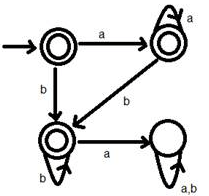Hence M-N equations = 4

QUESTION: 18

Suppose a processor uses a round robin scheduling algorithm to schedule the process. Previously, it was using a time slice of 2 units while scheduling the process. An update is made to the system and the time slice is now changed to 4 units. Then the turnaround time of the process will

Solution: For the process with small burst times, turnaround time will decrease but for the process with large burst time, it will increase. Hence we can say it varies according to the burst time of the process.
QUESTION: 19

Which of the following is/are true?

Solution:

1. Option A is false, because semaphores are the solution used to avoid busy waiting.

2. Option B is false, Since Priority inversion causes the problem of LiveLock and priority Inheritance is the solution for Live Lock.

3. Option C is true.

QUESTION: 20

To realise a 128x1 MUX , how many 4x1 MUX are required _____________

Solution:

128/4 = 32

32/4 = 8

8/4 = 2

2/4 = 0.5 ≅ 1

Total = 32 + 8 + 2 + 1 = 43

QUESTION: 21

Consider the following Relational Schema:

Sailors (sid: integer, sname: string, rating: integer, age: real)

Boats (bid: integer, bname: string, color: string)

Reserves (sid: integer, bid: integer, day: date)

Consider the Following Statements:

S1:SELECT S.sname

FROM Sailors S

WHERE NOT EXISTS (SELECT *

FROM Sailors S2

WHERE S2.age < 21 AND S.rating <= S2.rating )

S2: SELECT S.sname

FROM Sailors S

WHERE S.rating > ANY (SELECT S2.rating

FROM Sailors S2

WHERE S2.age < 21 )

Which of the following is true regarding S1 and S2?

Solution:

S1: it will generate the name of sailors whose rating is more than the rating of every sailor whose age is less than 21.

S2: it will generate the name whose rating is more than the rating of the same sailor with age less than 21.

QUESTION: 22

Consider a binary tree with the following more conditions :

Condition 1: The elements present in the left sub-tree of a specific node are smaller than that node and elements present in the right sub-tree of a specific node are greater than that node.

Condition 2: The tree is always balanced i.e. the height of the left subtree and right sub-tree is approximately the same. (maximum differ by 1)

What is the worst-case time complexity to run a membership test for an element on this tree?

Solution: The tree is nothing but a balanced BST where the worst case time to find an element = O(logn)
QUESTION: 23

Consider a lower triangular matrix. When this lower triangular matrix is stored in array format then only the elements a[i][j] with i≥j are stored in array i.e only the elements present in lower triangular matrix are stored. Hence less size is consumed to store the array. Consider a lower triangular matrix as [25----100 , 25----100] with base address as 1000 and size of each element in matrix is 10, If the array is stored in column major order then find the address of the element a  stored in array ________________.

Solution:

In general , if the array is a [lb1---ub1 , lb2----ub2]

Size of element = c

Number of rows = nr = ub1- lb1 + 1

Number of columns = nc = ub2 - lb2 + 1

and lower triangular matrix stored in column major order then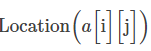=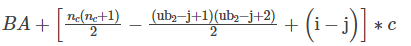So,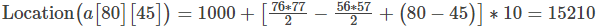QUESTION: 24

Suppose there are certain number of peoples who’s usage are ALOHA dependent and they are producing 100 requests/sec. Consider time to be slotted in 50msec units. After considering all of the above cases find out the chances of win on the 1st attempt?

Solution:

Number of slots per second = 1/50msec = 20

Channel load =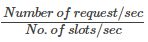= 100/20 = 5

We know, Poisson Distribution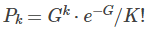or the success on the first attempt

K=0

P0 = e-G = e-5

= 6.73 x 10-3

QUESTION: 25

You have a class B network 172. 16. 0. 0. You use 11 bits for subnetting. Which of the following is a correct range of IP addresses that belong to the same network?

Solution:

If we use 11 bits for subnetting, we have 5 networking bits and 11 node bits

From the subnetting formulas above:

M = 5, N=11

The first range = 255.255.X.1 to 255.255.Y.254 where X = 2(N-8) + 1 and Y = 2(N-8-1) – 2

For the next ranges, just add 2(N-8) at each end of the range

The first range = 255.255.9.1 to 255.255.14.254 = 2(N-8)= 23=8

The second range = 255.255.17.1 to 255.255.22.254 so on and so forth.

QUESTION: 26

Consider the following relations, SQL query and given instances of relations: (where keys are underlined)

Student (snum, sname)

Enroll (snum, cname)

SELECT S.name FROM Student S WHERE

S.snum NOT IN (SELECT E.snum FROM Enroll E)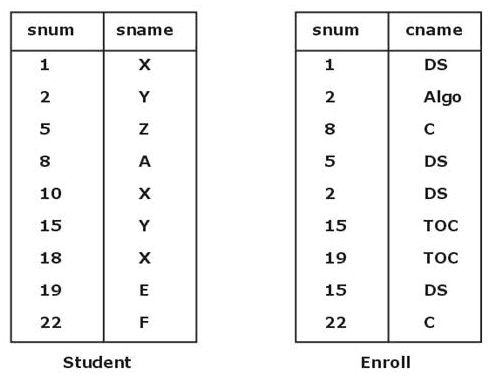Number of tuples returned by the SQL query is ________.

Solution:

SELECT S.Name FROM Student S WHERE

S.snum NOT IN (SELECT E.snum FROM Enroll E)

It return snum of all students who is enrolled in any course.

Query return the sname of a student who is not enrolled in any course.

SQL does not eliminates duplicate so relation given by SQL query is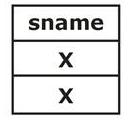Total 2 tuple returns.

QUESTION: 27

Consider two languages, L1and L2:

L1= {an| n > = 0} and L2 = {bn | n > = 0}

Which of the following correctly represents L1⋅ L2, where ‘⋅’ is the concatenation operation?

Solution: L1 . L2 will be equal to {an bm| m , n > = 0}, which is same as {an bm| m = n, n > –1}.
QUESTION: 28

Consider the following regular expressions over the alphabet {0, 1}.

I. 1* 0(0 + 1)*

II. (0 + 1)* 01*

III. 1* 0(1 + 0)* + (0 + 1)* 01*

Which of the above regular expressions are equivalent?

Solution: I and II are quite easy to understand - both denote strings containing at least one 0. III is actually the union of I and II, but III is again denoting strings containing at least one 0, as both regular expressions (i.e. I and II) are equivalent, so union won't make any difference.

Hence all 3 regular expressions are equivalent.

QUESTION: 29

A 4-bit preset table UP counter has preset input 0111. The preset operation takes place as soon as the counter becomes maximum, i.e. 1111. The modulus of this counter is

Solution: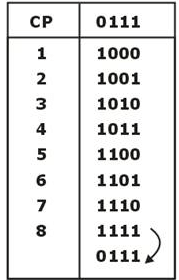Preset → 8 clock pulses, it is repeating

So mod 8.

QUESTION: 30

Let R be a recursive language. Consider the following operations on languages.

I. Union

II. Intersection

III. Complement

Let X be the number of operations under which R is closed. Then the value of X is ________.

Solution: Recursive languages are closed under all the above operations.
QUESTION: 31

Consider the following synchronization construct used by two process P0 and P1 which need to access a critical section:

P0 :

while (true) {

turn_0 = true;

while (turn_l = = true)

turn_0 = false;

CRITICAL SECTION

}

/ * Remainder section*/

P1 :

while (true) {

turn_1 = true;

while (turn_0 = = true)

turn_1 = false;

CRITICAL SECTION

}

/*Remainder section*/

Here, turn_0 and turn_1 are shared variables, which are initialized to false. Which of the following statements is true about the above construct?

Solution: When both processes P0 and P1 execute concurrently. P0 and P1 make turn_0 = true and turn_1 = true, process P0 executes while loop before executing turn_0 = false it preempts and P1 starts executing it to make tum_1 = false and preempted. P0 makes turn_0 = false, both processes enter into the critical section. It does not ensure mutual exclusion.
QUESTION: 32

Consider the following sentence:

“There is exactly one God”

Let G(x) : x is a God

Now consider the following predicate logic statements.

I. ∃ × God⁡(x)

II. ∃ × God⁡(x) ∧∀y (God(y) ⇒ x = y))

III. ∃ x ∀y(God⁡(x) ∧ (∼ God⁡(y) ∨ x =y))

The number of statements which are equivalent to the above sentence is ________.

Solution:

* I is not correct because it says "at least one God" instead of "exactly one God”.

* II is clearly the correct statement.

* III is same as II and can be obtained using,

p ⇒ q ≡ ∼ p ∨ q

\)God\y) \Rightarrow(x = y \equiv(\sim \operatorname{God}(y) \vee x = y\)

QUESTION: 33

A 3×3 matrix has 3 eigenvalues -1,0.5,3 . What will be the eigenvalues of P2 +2P + I?

Solution:

P2 + 2P + I can be written as,

= P2+ 2P.I + I

= (P + I)2

Eigen Values of I are (1, 1, 1)

Eigen Values of P are (-1, 0.5, 3)

Eigen Values of (P + I) will be (-1 + 1, 0.5 + 1, 3 + 1) = (0, 1.5, 4)

Eigen values of (P + I)2 will be (02, 1.52, 42) = (0, 2.25, 16) =

(0,9/4,16)Hence (A) is the most appropriate choice.

QUESTION: 34

Let (G, *) be a group such that O(G) = 8, where O(G) denotes the order of the group G. Which of the following is true?

Solution: Theorem: If a is an art element of G, then. O(A) must divide O(G).

Therefore (A) is false, as O(A) = 6 and 6 doesn’t divide 8.

However the converse of the above theorem need not be true, therefore (B) is false.

(C) says there are at least two elements in G with order 1, however this violates the fact that every group can have only one identity element. So (C) is also wrong. So the appropriate choice is (d).

QUESTION: 35

The number of one-one functions possible from a set having n elements to a set having m elements is

Solution: The number of one-one functions from n element set to m element set = mPn which is the same as mCn.n!

Hence option (d) is correct.

QUESTION: 36

Consider a computer system with 32-bit virtual addressing and 44-bit physical addressing and page size is 4 KB. Each page table entry contains 2 valid bits, 3 protection bits and 2 permission bits. Size of the page table when virtual memory uses single level paging ________ (MB).

Solution:

Number of pages =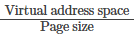= 232 / 212 = 220

Number of frame = 244 / 212 = 232

Number of bits in page table entry =32+2 valid bit +3 protection bit +2 permission bit =39 bit = 5B

Page table size = 220 x 5B = 5MB

*Answer can only contain numeric values
QUESTION: 37

Consider a 2-way set associative cache with total 6 cache blocks and the following sequence of memory block requests arrived:

21, 7, 20, 32, 21, 16, 27, 22, 7, 16, 22

If LRU replacement policy is used then the hit ratio will be ________. (Upto 2 decimal places.)

Solution: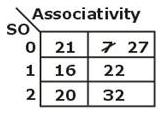21 mod 3 = 0 → miss

7 mod 3 = 1 → miss

20 mod 3 = 2 → miss

32 mod 3 = 2 → miss

21 mod 3 = 0 → hit

16 mod 3 = 1 → miss

27 mod 3 = 1 → miss

22 mod 3 = 1 → miss

7 mod 3 = 0 → miss

16 mod 3 = 1 → hit

22 mod 3 = 1 → hit

Hit Ratio = Total Hit / Total Reference = 3 / 11 = 0.27

QUESTION: 38

Consider the following relation R(A, B, C, D, E, F, G) and set of functional dependencies.

F = {BCD → A, BC → E, A → F, F → G, C → D, A → G}

Which of the following is the minimal cover of F?

Solution:

(1) RHS contain single attribute

A → F …..(1)

F → G …..(2)

A → G …….(3)

Hence FD' (3) is redundant.

Now consider

BCD → A

(BC)+ = BCEDAFG

It contains attribute D, D is redundant.

Minimal cover is {BC → A, BC → E, A → F, F → G, C → D}

So option (c) is correct.

QUESTION: 39

Assume that the time complexity of the most efficient algorithm to find the median in a list of n characters is θ(n logn). It is experimentally found that, when this algorithm is made to run on a list of 16 elements, it takes 256 units of time respectively. Then the time taken by this algorithm when the input list contains 64 elements is _______.

Solution:

Given, T(n) = θ(n logn) = c.nlog n

Now, T(16) = 256 units

Therefore, c.16.log (16) = 256

⇒ c = 4

Now we have to find T(64).

T(64) = 64.c.log (64)

Substitute the value of c and solve the equation to get

T(64) = 1536 units

QUESTION: 40

Consider the following graphs: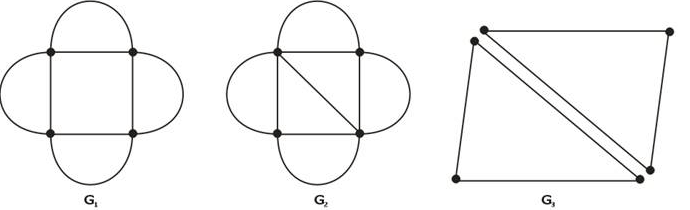The number of graphs which are Euler ________.

Solution:

For a graph to be Euler, it must obeys the following conditions:

1. Every vertex of G must have an even degree.

2. G should be connected.

G1 is Euler, as it is connected and all vertices in G1 have even degree.

G2 has 2 vertices with odd degree ⇒ G2 is not Euler.

G3 is not connected ⇒ G3 is not Euler.

QUESTION: 41

The cube root of a natural number n is defined as the largest natural number m such that m3 ≤ n. The complexity of computing the cube root of n (n is represented in binary notation) is:

Solution:

You need to find upper and perhaps lower bounds on the complexity of finding an integer cube root m of n. At least one upper bound is trivial, and rules out answers A and B: m can be found in O(log n) time using binary search.

Also note that the input size is O(log n) because the minimum number of bits needed to represent an arbitrary n in binary notation is proportional to log n. Because all bits of the number must be processed to solve the problem, θ(log n) is a lower bound on the time to solve the problem, and therefore the problem cannot be solved in time O((log log n)w) [where w is some constant > 0] because that isn't O(log n).

QUESTION: 42

For a complex number z, find the value of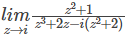Solution:

=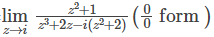Applying L’ Hospital rule

=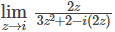=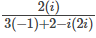=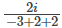= 2i

QUESTION: 43

Identify the language accepted by the following deterministic finite automata over the input alphabet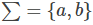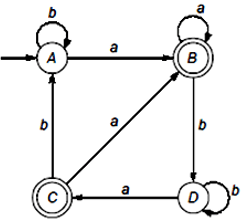Solution: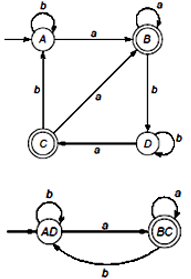Is equivalent to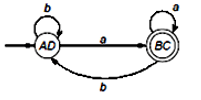The given DFA accepts the language of all strings where every string ends with a.

QUESTION: 44

The maximum number of edges in a bipartite graph on 12 vertices is_______.

Solution:

The number of edges in a bipartite graph on n-vertices is atmost n2/4

The maximum number of edges in a bipartite graph on 12 –vertices is n2/4 = 12 x 12 / 4 = 36

QUESTION: 45

Which of the following helps in conversion of deterministic finite automata into a regular expression?

Solution:

Given a DFA, Kleene’s algo helps to convert it into regular expression. Floyd-Warshall algorithm is out of context because it is used in graphs and Thompson’s Construction Algorithm transforms a regular expression into an equivalent NFA.

QUESTION: 46

In a 8 bit SISO register, if 16-bit data 1011 0101 0010 1010 is applied at the input. The minimum number of clock pulses required to transfer 16-bit data at the output is.

Solution:

Given that 8 bit SISO register n = 8, input data = N = 6 bits.

After application of 16th clock pulse out of 16-bit data 8 will already transferred and remaining 8 will be inside register, so to transfer these 8 we need 7 more clock pulse, so total = 16 + 7 = 23

Minimum number of clock pulse=N + (n -1) = 16 + 8 - 1 = 23

QUESTION: 47

A transaction state changes from active to after the transaction has been rolled back and the database restored to its state prior to the start of the transaction

Solution:

A transaction state changes from active to aborted, after the transaction has been rolled back and the database restored to its state prior to the start of the transaction.

QUESTION: 48

An instruction is stored at location 300 with its address field at location 301. The address field has the value 400. A processor register R1 contains the number 200. What will be the addressing mode of the effective address of the instruction is 702?

Solution:

Relative: 302 + 400 = 702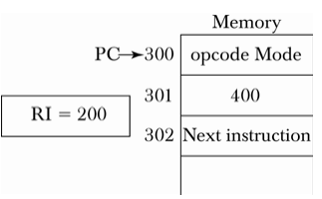In this mode the content of the program counter is added to the address part of the instruction in order to obtain the effective address.

QUESTION: 49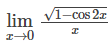is__________

Solution: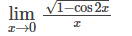=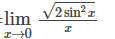=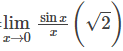Using L' Hospital rule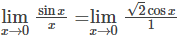= √2 = 1.414

QUESTION: 50

The difference between local maximum and local minimum value of f(x)=2x3 - 24x + 107is

Solution:

f(x)=2x3 − 24x + 107

⇒ f′(x) = 6x2 − 24=0

⇒ x = ±2 are stationary points

f′′(x) = 12x

⇒ f′′(2) = 24>0, 0,therefore,local minima

f′′(−2) = −24>0, 0,therefore,local minima

f(−2) = 2(−2)3 + 48 + 107 = 139

f(2) = 2(2)3 − 48 + 107 = 75

∴ Difference = f(−2) - f(2) = 64

QUESTION: 51

Regular languages are

Solution:

According to Chomsky hierarchy, regular languages come under type 3 i.e. level 3 language class.

QUESTION: 52

Which of the following is correct output for the program code given below?

main( )

{

void pr( );

pr ( );

pr ( );

pr ( );

void pr ( )

{

static int i = 1;

printf (“%c”, (65+ i ++));

}

}

Solution:

The correct output is “BCD” when the function pr ( ) is first called the value of i is initialized to 1.

After the pr ( ) completes its execution i = 2 is retained for it’s next call as “i” is a static variable.

∴ 65 + 1 = 66 = B

65 + 2 = 67 = C

65 + 3 = 68 = D

∴ BCD is the correct output.

QUESTION: 53

A survey of 150 college students reveals that 83 own cars, 97 own bikes, 28 own motorcycles, 53 own a car and a bike, 14 own car and a motorcycle, 7 own a bike and a motorcycle and 2 own all three. How many students own a bike and nothing else?

Solution:

A→ The set of students owning cars B→ The set of students owning bikes C→ The set of students owning motorcycles. |A| = 83, |B| = 97, |C|=28, |A ∩ B| = 53

|A∪C| = 14, |B∩C| = 7, |A ∩ B ∩ C| = 2

|A ∪ B ∪ C|=150

(B + ABC − AB − BC) denotes the set of students who owns bike and nothing else

QUESTION: 54

If s is a string over (0+1)∗ then let n0(s) denote the number of 0 's in sand n1(s) the number of 1 's in s. Which one of the following languages is not regular?

Solution:

a) 3 digit primes are finite, hence regular

b) Only finite combinations, hence regular

c) L={s∈(0+1)∗|n0(s)−n1(s)|≤4} is not regular because to keep track of

a number of 0 stacks is required.

d) regular, it is a standard mod machine

QUESTION: 55

Let X be a random variable following normal distribution with mean +1 and variance 4. Let Y be another normal variable with mean –1 and variance unknown. If P(X ≤ -1) = P(Y ≥ 2), the standard deviation of Y is

Solution:

X(,4),4(−1,σ2)

P(X ≤ −1)

Z = −1 − 1 / 2 = −1

\( = P(Z \geq-1) = P(Z \geq 1) = 0.5 - P(0 = 0.5 − 0.3413 = 0.1587

If σ = 3 then

P(Z ≥ 2),Z = 2 + 13 = 1

QUESTION: 56

f(x,y)=tan(y3/x3)y2x2 +xy3+y4

What is the value of fx+2fy at point(3,6)

Solution:

Given the function f(x,y) is a homogeneous function,so can be applied Euler’s theorem.

Applying Euler’s theorem on f(x,y),we get

xfx + yfy = nf(x, y) [n =4 here]

Substituting (x,y)=(3,6) we have

3fx + 6fy = 4f(3,6) =4(tan(216/27)36 × 9 + 3 × 216 + 216 × 6)

fx+2fy=4(108tan(216/27)+648)

=432 tan (8) + 2592.

QUESTION: 57

A disk has 200 tracks(from 0 to 199). At time t = 0, the pending requests for read are for the following disks in the order:

46, 110, 32, 52, 14, 120, 36, 96.

Current head position is at 50 and moving towards 0.

The total head movement(in number of tracks) incurred if LOOK is applied is

Solution:

If LOOK is applied, the requests are served in the order: 46, 36, 32, 14, 52, 96, 110, 120

But it moves from 14 to 52 without moving upto 0 and then to 52.

Total track movements = 10+4+18+(52−14)+96−52+110−96+10 = 138.

QUESTION: 58

Consider the following arguments about Bankers algorithm.

i. Each process must have apriori claim of its maximum requirement

ii. There are multiple instances of each resource type

iii. It is a deadlock prevention algorithm

iv. It is a resource allocation algorithm

Which of the following are true?

Solution:

Bankers algorithm is a deadlock avoidance algorithm. Requires that the system has some additional a priori information available.

Simplest and most useful model requires that each process declares the maximum number of resources of each type that it may need. The deadlock-avoidance algorithm dynamically examines the resource-allocation state to ensure that there can never be a circular-wait condition.

Resource-allocation state is defined by the number of available and allocated resources, and the maximum demands of the processes. So except statement iii, all are true.

QUESTION: 59

A disk has 200 tracks(from 0 to 199). At time t = 0, the pending requests for read are for the following disks in the order:

46, 110, 32, 52, 14, 120, 36, 96.

Current head position is at 50 and moving towards 0.

If the SSTF algorithm and SCAN algorithm are used, difference in total movement of tracks is

Solution:

In case of SSTF algorithm, the requests are served in this order

52, 46, 36, 32, 14, 96, 110, 120⇒Total track movements = 146

In case of SCAN, the requests are served in the order: 46, 36, 32, 14, 52, 96, 110, 120

Total track movements = (46-36)+(36-32)+(32-14)+(14-0)+(52-0)+(96-52)+(110-96)+(120-110) = 170.

Hence extra movement =20.

QUESTION: 60

A system uses FIFO policy for page replacement. It has 4 page frames with no pages loaded to begin with. The system first accesses 50 distinct pages in some order and then accesses the same 50 pages in reverse order. How many page faults will occur?

Solution:

50 distinct pages(forward) - 50 page replacements

Reverse order- first 4 pages will be already loaded- then remaining 46 page replacements Total - 96.

QUESTION: 61

Let L1 be a regular language, L2 be a deterministic context-free language and L3 a recursively enumerable but not recursive language.

Which one of the following statements is false?

Solution:

A) L1∩L2 is a deterministic CFL, because Regular ∩ DCFL is

always DCFL

B) L3∩L1 is need not be recursive, But always REL

C) L1.L2 is context free, because Regular. DCFL is always DCFL

D) L1∩L2∩L3 is recursively enumerable, because all are REL

QUESTION: 62

Let f be the fraction of a computation (in terms of time) that is parallelizable, P the number of processors in the system, and sp the speed up achievable in comparison with sequential execution – then the sp can be calculated using the relation:

Solution:

If 'f' be the fraction of a computation that can be parallelized than speedup =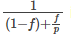is the relation that can be used to calculate speedup.

QUESTION: 63

Consider a problem Z, in it-

“Suppose there is a Turing Machine L over the input alphabet ∑ any state of L and a word s € ∑, does the computation of L on s visit the state q?

Choose the correct statement about Z?

Solution:

This problem is a State Entry Problem. State entry problem can be reduced to a halting problem. We construct a turing machine L with final state ‘q’. We run a turning machine E (for state entry problem) with inputs : L, q, s. We give ‘s’ as input to L. If L halts in the final state ‘q’ then E accepts the input. So, the given problem is partially decidable. If L goes in an infinite loop then L can not output anything. So, E rejects the input. So, the given problem becomes undecidable.

QUESTION: 64

Suppose Suresh and Aditya are shown combinely P1 is called the 3 -SAT problem. Which of the following is correct?

Solution:

P1 is 3 -SAT problem and also NPC-problem. P1 is solvable in polynomial time.

Now, the 3-SAT problem is a P-problem.

⇒ P = NPC

⇒ P = NP = NPC

∴ Option B is correct.

QUESTION: 65

Which one of the following is not involved in Preventing the dead lock

Solution:

To prevent deadlock, at least one of the following conditions cannot occur.

• Mutual exclusion.

• Hold and wait

• No preemption

• Circular wait.

No “mutual exclusion “, No '' Hold and wait”, preemption or no circular wait condition occurs to prevent deadlock.Use Code STAYHOME200 and get INR 200 additional OFF Use Coupon Code